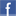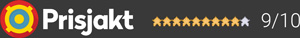#### Inga produkter tillagda!

Lägg en produkt i varukorgen så ser det lite roligare ut här ;)

#### Varukorg

0 kr# ArcamSA20

13 300 krLeverans 2-5 dagar ()
Fri frakt!

SA20

There are two frequent problems with hi-fi amplifiers. Class A, the traditional audiophile’s choice, uses a single power supply constantly running at full capacity, even when there’s no signal coming through. Great for reproducing those subtle details at low levels however struggles to provide extreme power when needed.

Class A/B, the most common amp class, switches between the two internal amplifiers, one for each half of the waveform. Far more efficient but often a recipe for crossover distortion, since those low-level details we crave can get lost within any mis-match between the two switching over.

Class G is essentially a hybrid amplifier, utilising Class A specifically for low-level signals, but when required switching in an additional power supply to power those larger sections of the waveform.

Garanti i 24 månaderDela på Facebook

#### Frågor? Ring 031-241600eller maila //<![CDATA[ var l=new Array(); l = '>'; l = 'a'; l = '/'; l = '<'; l = '|109'; l = '|111'; l = '|99'; l = '|46'; l = '|110'; l = '|114'; l = '|97'; l = '|108'; l = '|108'; l = '|97'; l = '|107'; l = '|100'; l = '|117'; l = '|106'; l = '|108'; l = '|64'; l = '|111'; l = '|102'; l = '|110'; l = '|105'; l = '>'; l = '"'; l = '|109'; l = '|111'; l = '|99'; l = '|46'; l = '|110'; l = '|114'; l = '|97'; l = '|108'; l = '|108'; l = '|97'; l = '|107'; l = '|100'; l = '|117'; l = '|106'; l = '|108'; l = '|64'; l = '|111'; l = '|102'; l = '|110'; l = '|105'; l = ':'; l = 'o'; l = 't'; l = 'l'; l = 'i'; l = 'a'; l = 'm'; l = '"'; l = '='; l = 'f'; l = 'e'; l = 'r'; l = 'h'; l = ' '; l = 'a'; l = '<'; for (var i = l.length-1; i >= 0; i=i-1) { if (l[i].substring(0, 1) === '|') document.write("&#"+unescape(l[i].substring(1))+";"); else document.write(unescape(l[i])); } //]]>